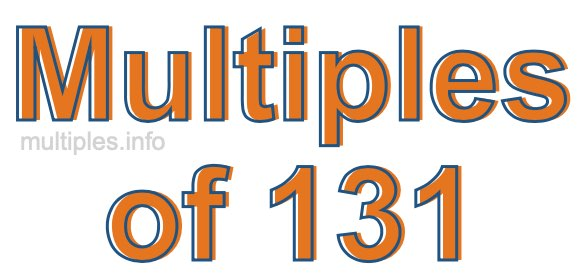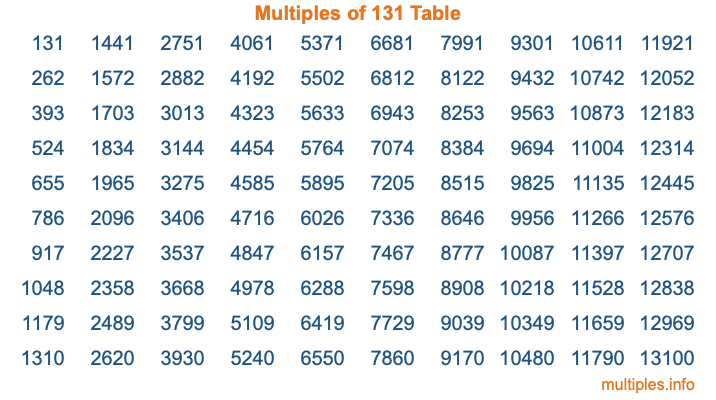Multiples of 131Welcome to the Multiples of 131 page. Here we will first teach you everything you will ever need to know about the multiples of 131, and then give you a study guide summary of everything we taught you to make sure you remember it all. Use this page to look up facts and learn information about the multiples of 131. This page will make you a multiples of one hundred thirty-one expert!

Definition of Multiples of 131
Multiples of 131 are all the numbers that when divided by 131 equal an integer. Each of the multiples of 131 are called a multiple. A multiple of 131 is created by multiplying 131 by an integer.

Therefore, to create a list of multiples of 131, you start with 1 multiplied by 131, then 2 multiplied by 131, then 3 multiplied by 131, and so on for as long as you want. Thus, the list of the first five multiples of 131 is 131, 262, 393, 524, and 655. To see a larger list of multiples of 131, see the printable image of Multiples of 131 further down on this page. We also have a category where you can choose any nth multiple of 131.

Multiples of 131 Checker
The Multiples of 131 Checker below checks to see if any number of your choice is a multiple of 131. In other words, it checks to see if there is any number (integer) that when multiplied by 131 will equal your number. To do that, we divide your number by 131. If the the quotient is an integer, then your number is a multiple of 131.

Is  a multiple of 131?

Least Common Multiple of 131 and ...
A Least Common Multiple (LCM) is the lowest multiple that two or more numbers have in common. This is also called the smallest common multiple or lowest common multiple and is useful to know when you are adding our subtracting fractions. Enter one or more numbers below (131 is already entered) to find the LCM.

Check out our LCM Calculator if you need more details about the Least Common Multiple or if you need the LCM for different numbers for adding and subtraction fractions.

nth Multiple of 131
As we stated above, 131 is the first multiple of 131, 262 is the second multiple of 131, 393 is the third multiple of 131, and so on. Enter a number below to find the nth multiple of 131.

th multiple of 131

Multiples of 131 vs Factors of 131
131 is a multiple of 131 and a factor of 131, but that is where the similarities end. All postive multiples of 131 are 131 or greater than 131. All positive factors of 131 are 131 or less than 131.

Below is the beginning list of multiples of 131 and the factors of 131 so you can compare:

Multiples of 131: 131, 262, 393, 524, 655, etc.

Factors of 131: 1, 131

As you can see, the multiples of 131 are all the numbers that you can divide by 131 to get a whole number. The factors of 131, on the other hand, are all the whole numbers that you can multiply by another whole number to get 131.

It's also interesting to note that if a number (x) is a factor of 131, then 131 will also be a multiple of that number (x).

Multiples of 131 vs Divisors of 131
The divisors of 131 are all the integers that 131 can be divided by evenly. Below is a list of the divisors of 131.

Divisors of 131: 1, 131

The interesting thing to note here is that if you take any multiple of 131 and divide it by a divisor of 131, you will see that the quotient is an integer.

Multiples of 131 Table
Below is an image of the first 100 multiples of 131 in a table. The table is in chronological order, column by column. The first column has the first ten multiples of 131, the second column has the next ten multiples of 131, and so on.The Multiples of 131 Table is also referred to as the 131 Times Table or Times Table of 131. You are welcome to print out our table for your studies.

Negative Multiples of 131
Although not often discussed or needed in math, it is worth mentioning that you can make a list of negative multiples of 131 by multiplying 131 by -1, then by -2, then by -3, and so on, to get the following list of negative multiples of 131:

-131, -262, -393, -524, -655, etc.

Multiples of 131 Summary
Below is a summary of important Multiples of 131 facts that we have discussed on this page. To retain the knowledge on this page, we recommend that you read through the summary and explain to yourself or a study partner why they hold true.

There are an infinite number of multiples of 131.

A multiple of 131 divided by 131 will equal a whole number.

131 divided by a factor of 131 equals a divisor of 131.

The nth multiple of 131 is n times 131.

The largest factor of 131 is equal to the first positive multiple of 131.

131 is a multiple of every factor of 131.

131 is a multiple of 131.

A multiple of 131 divided by a divisor of 131 equals an integer.

131 divided by a divisor of 131 equals a factor of 131.

Any integer times 131 will equal a multiple of 131.

Multiples of a Number
Here you can get the multiples of another number, all with the same attention to detail as we did for multiples of 131 on this page.

Multiples of
Multiples of 132
Did you find our page about multiples of one hundred thirty-one educational? Do you want more knowledge? Check out the multiples of the next number on our list!